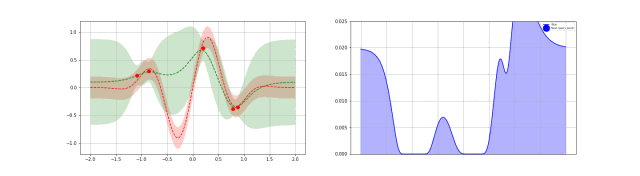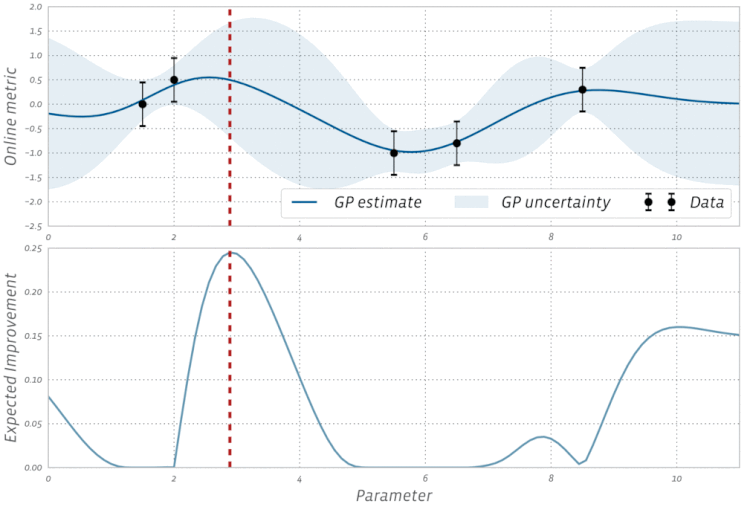# ggaaooppeenngg

## 贝叶斯优化(BO)

BayesOpt consists of two main components: a Bayesian statistical model for modeling the objective function, and an acquisition function for deciding where to sample next.

## 高斯过程(GP)Definition: A GP is a (potentially infinte) collection of random variables (RV) such that the joint distribution of every finite subset of RVs is multivariate Gaussian:
$f \sim GP(\mu, k),$
where $\mu(\mathbf{x})$ and $k(\mathbf{x}, \mathbf{x}’)$ are the mean resp. covariance function! Now, in order to model the predictive distribution $P(f_* \mid \mathbf{x}_*, D)$ we can use a Bayesian approach by using a GP prior: $P(f\mid \mathbf{x}) \sim \mathcal{N}(\mu, \Sigma)$ and condition it on the training data $D$ to model the joint distribution of $f=f(X)$ (vector of training observations) and $f_* = f(\mathbf{x}_*)$ (prediction at test input).

## 采样函数

Explore：探索新的点，这种采样有助于估计更准确的；
Exploit：利用已有结果附近的点进行采样，从而希望找到更大的；

Expected improvement is a popular acquisition function owing to its good practical performance and an analytic form that is easy to compute. As the name suggests it rewards evaluation of the objective $f$ based on the expected improvement relative to the current best. If $f^* = \max_i y_i$ is the current best observed outcome and our goal is to maximize $f$, then EI is defined as

$\text{EI}(x) = \mathbb{E}\bigl[\max((f(x) - f^*), 0)\bigr]$

BoTorch 提供了一个优化的 EI 叫 Noisy EI，主要功能是抗噪。

The above definition of the EI function assumes that the objective function is observed free of noise. In many types of experiments, such as those found in A/B testing and reinforcement learning, the observations are typically noisy. For these cases, BoTorch implements an efficient variant of EI, called Noisy EI, which allow for optimization of highly noisy outcomes, along with any number of constraints (i.e., ensuring that auxiliary outcomes do not increase or decrease too much). For more on Noisy EI, see our blog post.## 应用

Horovod comes with several adjustable “knobs” that can affect runtime performance, including –fusion-threshold-mb and –cycle-time-ms (tensor fusion), –cache-capacity (response cache), and hierarchical collective algorithms –hierarchical-allreduce and –hierarchical-allgather.